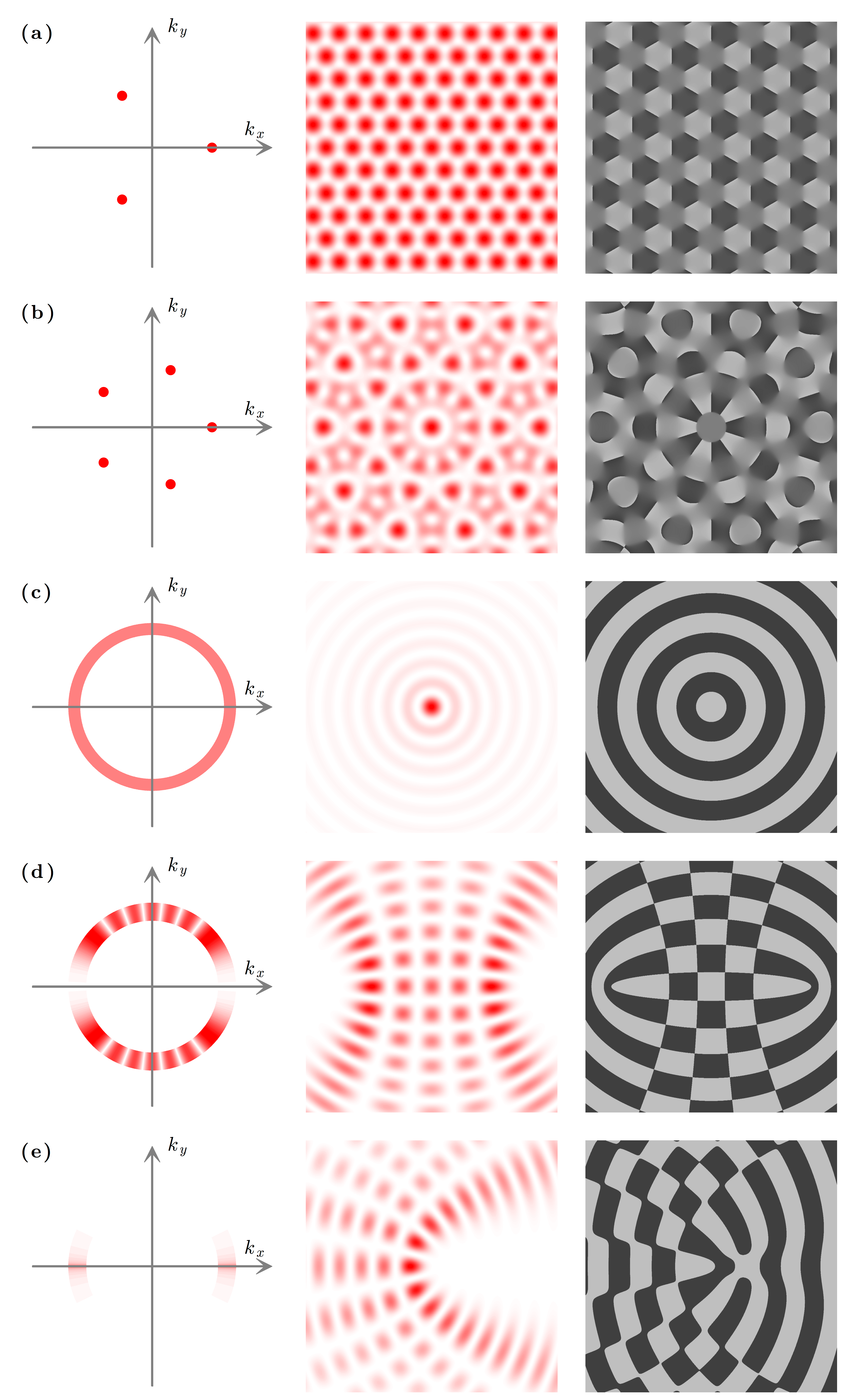# Figure 4.9 — Non-diffracting beamsFigure 4.9 — Non-diffracting beams. (a) Periodic hexagonal and (b) quasiperiodic fivefold tilings produced by discrete beams with m = 0 [Eq. (4.31)]. (c) Bessel beam with m = 0 [Eq. (4.32)]. (d) Even Mathieu beam with m = 4 and q = 6.25π 2 [Eq. (4.33)]. (e) Even Weber beam with a = −2 [Eq. (4.35)]. From left to right, the columns represent the distribution of the wavevectors, the intensity and the phase.